# Plus One Physics Chapter Wise Questions and Answers Chapter 7 Systems of Particles and Rotational Motion

Students can Download Chapter 7 Systems of Particles and Rotational Motion Questions and Answers, Plus One Physics Chapter Wise Questions and Answers helps you to revise the complete Kerala State Syllabus and score more marks in your examinations.

## Kerala Plus One Physics Chapter Wise Questions and Answers Chapter 7 Systems of Particles and Rotational Motion

### Plus One Physics Systems of Particles and Rotational Motion One Mark Questions and Answers

Plus One Physics Chapter 7 Systems of Particles and Rotational Motion Question 1.
The dimension of angular momentum is
(a) M°L1T-1
(b) M1L2T2
(c) M1L2T-1
(d) M2L1T-2
(c) M1L2T-1
Angular momentum = Moment of inertia x angular velocity
(Angular momentum) = [M1L2][T-1] = [M1L2T-1].

Plus One Physics System Of Particles And Rotational Motion Question 2.
If the density of material of a square plate and a circular plate shown in figure is same, the centre of mass of the composite system will be(a) inside the square plate
(b) inside the circular plate
(c) at the point of contact
(d) outside the system
(a) inside the square plate

Plus One Physics Important Questions And Answers Pdf Question 3.
Why spokes are provided in by cycle wheel?
This increases moment of inertia even when the mass is small. This ensures uniform speed.

Plus One Physics Chapter Wise Questions And Answers Question 4.
A ballet dancer, an acrobat and an ice skater make use of an important principle in physics. Which is that principle?
Conservation of angular momentum.

Physics Class 11 Chapter 7 Important Questions Question 5.
A cat is able to land on her feet after a fall. Which principle of physics is being used by her?
Principle of conservation of angular momentum.

Hsslive Plus One Physics Chapter Wise Questions And Answers Question 6.
A body is rotating in steady rate. What is torque acting on the body?
Zero. Torque is required only for producing angular acceleration.

Plus One Physics Chapter Wise Questions And Answers Pdf Hsslive Question 7.
A flywheel is revolving with constant angular velocity. A chip of its rim breaks and flies away. What will be the effect on the angular velocity?
The reduction in mass will decrease moments of inertia. Hence angular velocity will be increased in order to conserve angular momentum.

Rotational Motion Class 11 Important Questions Question 8.
Is radius of gyration of a body constant quantity?
No. It changes with change in position of the axis of rotation.

System Of Particles And Rotational Motion Class 11 Solutions Question 9.
What is another name for angular momentum?
Moment of momentum.

### Plus One Physics Systems of Particles and Rotational Motion Two Mark Questions and Answers

Hss Live Plus One Physics Chapter Wise Questions And Answers Question 1.
Moments of inertia of some bodies with axis are given in the table below. Fill in the blanks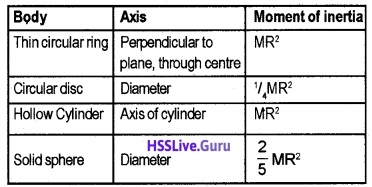System Of Particles And Rotational Motion Questions And Answers Question 2.
Match the following:

 (a) Moment of force Τ ∆ θ (b) F∆r Linear motion (c) Couple Torque (d) 1/2 Iω2 Τ ∆ r Rotational motion 1/2 MR2 L2/2I

(a) Torque
(b) τ ∆ θ
(c) Rotational motion
(d) $$\frac{1}{2} \frac{L^{2}}{1}$$.

System Of Particles And Rotational Motion Important Questions Question 3.
A cat is able to function its feet after a fall, taking the advantage of principle of conservation of angular momentum.

1. Give the law of conservation of a angular momentum.
2. Explain how cat is able to do so

1. When there is no external torque, the total angular momentum of a body or a system of bodies are a constant.
τ = $$\frac{d L}{d t}$$ (when τ = 0 , we get $$\frac{d L}{d t}$$ = 0).
ie L = constant.
But L = Iω
∴ Iω = a constant.

2. When a cat falls, it stretches its body. So that the moment of inertia becomes large. As Iω = constant, the value of angular speed will be decreased due to the increased value of moment of inertia. So cat lands on its feet gently.

System Of Particles And Rotational Motion Class 11 Important Questions Question 4.
If the polar ice cap melts what will happen to the length of the day?
For earth, angular momentum is a constant (Lω = constant, ie no torque acts on the earth). When the polar ice cap melts, the water thus formed will flow down to the equatorial region.

The accumulation of water in equatorial line will increase the moment of inertia I of earth. In order to keep the angular momentum as a constant, ω will decrease. The decrease in ‘ω’will increase the length of day.

System Of Particles And Rotational Motion Class 11 Question 5.
A girl has to lean towards right when carrying a bag in her left hand. Why?
When a girl carries her bag in her left hand, the centre of gravity of system will shift towards left. In order to bring it in the middle, the girl has to lean towards right.

Question 6.
If the earth loses the atmosphere what will happen to the length of the day?
For earth, the angular momentum (L = Iω) is a constant, because there is no torque acting on it. When earth loses the atmosphere, I decreases and ω increases to keep L as constant. Hence length of the day decreases.

Question 7.
A girl standing on a turn table. What happens to the rotation speed, if she stretches her hand?
lf a girl rotating with a uniform speed on turn table, it’s angular momentum (L = Iω) will be a constant. When she suddenly stretches her hand, I Increases and ω decreases to keep L as constant.

Question 8.
How does a circus acrobat and a diver take advantage of conservation of angular momentum? Answer:
The diver while leaving the spring board, is throwing himself in a rotating motion. When he brings his hands and legs close, I decrease and ω increases. But before reaching water he will stretch his hands and legs. Hence I increases and ω decreases. So, that he gets a smooth entry into the water.

### Plus One Physics Systems of Particles and Rotational Motion Three Mark Questions and Answers

Question 1.
A rigid body consists of ‘n’ particles of mass m1, m2, m3,……The body rotates about an axis with
an angular velocity ω1, ω2, ω3……..

1. Starting from the kinetic energy of a single particle, arrive at an equation for kinetic energy of rotation.
2. Moment of inertia is also called rotational inertia. Why?

1. Consider a body rotating about an axis passing through some point O with uniform angular velocity ‘ω’. The body can be considered to be made up of a number of particles of masses m1, m2, m3……etc at distances r1, r2, r3……etc. All the particles will have same angular velocity ω But their linear velocities will be different say v1, v2, v3…….etc.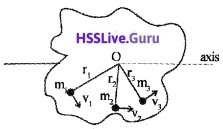K.E of 1st particle = $$\frac{1}{2}$$m1v12
$$\frac{1}{2}$$m1(r1ω)2
(∵ v = rω)
K.E of IInd particle = $$\frac{1}{2}$$m2(r2ω)2
∴ K.E of whole body =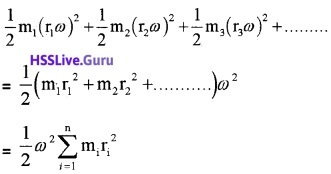But we know moment of inertia,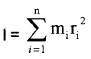∴ KE = $$\frac{1}{2}$$Iω2
2. Rotation inertia is measured in terms of moment of inertia. Hence moment of inertia is also called rotational inertia.

Question 2.
The handle of a door is always found at one edge of the door which is located at a maximum possible distance away from hinges.

1. Give reason for it.
2. In which direction will the torque act while the door opens inside the room?
3. If the door handle is fixed at the middle of the door, what difference do you feel in the applied force to open the door.

1. Torque τ = r F sin θ
From the above equation it is clear that, we get maximum torque when the handle of a door is located at a maximum possible distance (r) away from hinge.

2. The direction of torque is always along the axis of rotation of door.

3. If the door handle is fixed at middle, more force must be applied to get maximum torque that is required to open the door.

Question 3.
Moment of inertia depends on the mass, axis of rotation and distribution of mass of the body.

1. What are moment of inertia and radius of gyration?
2. How will you distinguish a hard boiled egg from a raw egg by spinning each on the table.

1. Moment of inertia I = mr2
Radius of gyration K = $$\sqrt{\frac{I}{m}}$$.

2. A raw egg has more monemt of inertia than boiled egg. Hence raw egg spins more time than boiled egg.

Question 4.
Table below given analogy between translational and rotational motions. Match the following.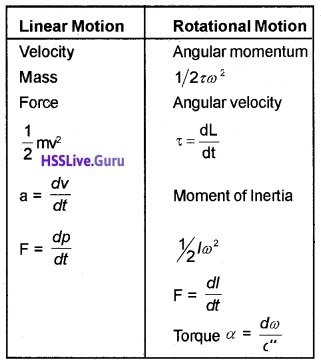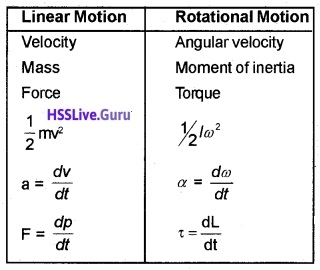### Plus One Physics Systems of Particles and Rotational Motion Four Mark Questions and Answers

Question 1.
1. Show that the total angular momentum of a rotating system remains constant if no torque acts on the system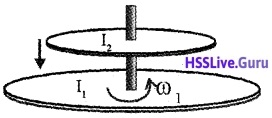2. A disc of moment of inertia I1 is rotating freely with angular speed ω1 and a second non rotating disc with moment of inertia I2 is dropped on it as shown in the figure. The two then rotate as one unit. Find the angular speed of rotation of the system.
1. we know torque τ = $$\frac{d L}{d t}$$
if τ = 0, we get $$\frac{d L}{d t}$$ = 0
ie. L = constant.

2. we know if torque acting on the body is zero, its angular momentum will be conserved
ie. I1ω1 = I2ω2
angular momentum of system, ω2 = $$\frac{I_{1} \omega_{1}}{\left(I_{1}+\mathrm{I}_{2}\right)}$$.

Question 2.
A rigid body can rotate an axis with a constant angular velocity and angular momentum L.

1. What is its moment of inertia about the axis?
2. Obtain a mathematical expression for rotational kinetic energy.
3. If the orientation of the axis of rotation changes, what happens to its moment of inertia

1. L = Iω
ie. I = L/ω

2. Consider a rigid body rotating about an axis passing through the point O. Let co be the uniform angular velocity of the body.The body is imagined to be made up of large number of particles. Consider one such particle of mass ‘m’ at a distance ‘r’ from the axis of rotation.
Linear Velocity of the particle v = rω
K.E of the particle = 1/2mv2 = 1/2 mr2ω2
K.E of whole body = Σ1/2mr2ω2 = 1/2ω2Σmr2
K.E = 1/2Iω2
Where Σmr2 = I, moment of inertia of the body.

3. Moment of inertia will be changed.

Question 3.
A platform diver holds his hands and legs straight and makes loops in air before entering into water.

1. State the principle behind this.
2. What happens when he tries to land in the pool by stretching his arms and legs?
3. In the above situation, rotational kinetic energy is not conserved. Explain.

1. Conservation of angular momentum
Statement
Conservation of angular momentum states that, if the total torque acting on a system is zero, its angular momentum will be conserved.

2. Angular velocity decreases.

3. Initial kinetic energy K.E1 = $$\frac{L^{2}}{2 I_{1}}$$ ____(1)
where I1 is the moment of inertia of diver when he makes loops in air
final kinetic energy K.E2 = $$\frac{L^{2}}{2 I_{2}}$$ _____(2)
where I2 is the moment of inertia of diver when he stretches his hands
But I1 < I2
Hence from eq(1) and eq(2), we get
KE1 > KE2
which means that rotational kinetic is not conserved.

Question 4.
Moment of inertia of a thin ring of radius R about an axis passing through any diameter is 1/2MR2

1. What is the radius of gyration of the ring about an axis passing through any diameter.
2. A thin metal ring of radius 0.25m and mass 2kg starts from rest and roll down an inclined plane. If the linear velocity on reaching the foot of the plane is 2m/s, calculate its rotational kinetic energy at that instant.

1.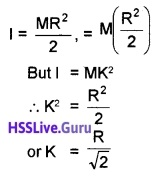2.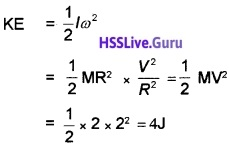Question 5.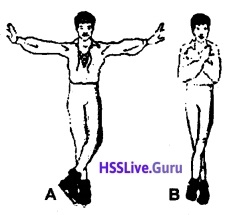Figures show the two different spinning poses of a ballet dancer.

1. In which spinning pose, the ballet dancer has more angular speed?
2. State the principle used by the ballet dancer to increase his angular speed.
3. “In the above situation, rotational kinetic energy is not conserved” – Justify this statement.

1. The pose shown in figure (B).

2. Statement of conservation of angular momentum.

3. I1ω1 = I2ω2
1/2 I12ω12 = 1/2 I22ω22
I1(1/2 I1ω12) = (1/2 I2ω22)I2, I1 > I2
(1/2 I2ω22) > 1/2I1ω12

### Plus One Physics Systems of Particles and Rotational Motion Five Mark Questions and Answers

Question 1.
The moment of inertia of a thin ring of radius R about an axis passing through any diameter is $$\frac{1}{2}$$MR2

1. To find the moment of inertia of the same ring about an axis passing through its centre of mass and perpendicular to its plane, which of the following theorem is used and state the theorem.
• Perpendicular axis theorem.
• Parallel axis theorem
2. What is the radius of gyration of the ring about an axis passing through its centre of mass and perpendicular to its plane?
3. A thin metal ring has a diameter 0.20 cm and mass 1 kg. Calculate its moment of inertia about an axis passing through any tangent.

1. perpendicular axis theorem.

2. Moment of inertia of ring,
I = mr2 ____(1)
Moment of inertia of ring in terms of radius of gyration,
I = mk2 ____(2)
From eq(1) and eq(2), we get
mk2 = mr2
radius of gyration, k = r.

3. I = I0 + ma2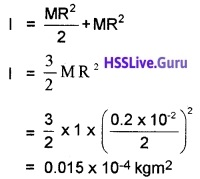Question 2.
The earth is moving around the sun in an elliptical orbit and this brings out the seasons.

1. Name the physical quantity conserved in this.
2. As the earth approaches near the sun in its path, it moves faster. State whether this statement is correct or wrong. Why?
3. Determine the duration of the day on earth if it suddenly shrinks to 1/4th of its present size while the mass of the earth remains unchanged. (Assume earth is a perfect sphere)

1. Conservation of angular momentum.

2. This statement is correct.
When earth approaches near the sun, its moment of inertia decreases. To maintain angular momentum as constant, the angular velocity increases.

3. New volume of earth = 1/4 original volume of earth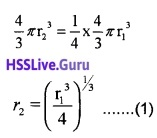Total angular momentum before shrinking = Total momentum after shrinking.
ie. I1ω1 = I1ω2
mr12ω1 = m22ω2 ______(2)
Sub eq(1) in eq(2)we know periodT2 = 3 hr
Duration of day T2 = 3 hr.

Question 3.
1. Find the moment of inertia of a sphere about a tangent to the sphere, given the moment of inertia of the sphere about any of its diameters to be 2MR2/5, where M is the mass of the sphere and R is the radius of the sphere.
2. Given the moment of inertia of a disc of mass M and radius R about any of its diameters to be MR2/4, find its moment of inertia about an axis normal to the disc and passing through a point on its edge.
1. Applying theorem of parallel axes, moment of inertia of sphere about a tangent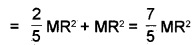2. Using theorem of perpendicular axis, the moment of inertia of the disc about an axis passing through the centre of the disc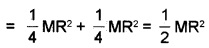Using theorem of parallel axes, moment of inertia of the disc passing through a point on its edge and the normal to the discQuestion 4.
A solid cylinder of mass 20kg rotates about its axis with angular speed 100rad s-1. The radius of the cylinder is 0.25m. What is the kinetic energy associated with the rotation of the cylinder? What is the magnitude of the angular mementum of the cylinder about its axis?
M = 20kg
angularspeed, co – 100 rad s-1; R = 0.25m
Moment of inertia of the cylinder about its axis
= $$\frac{1}{2}$$MR2 = $$\frac{1}{2}$$ × 20 × (0.25)2kgm2 = 0.625kgm2
Rotational kinetic energy,
Er = $$\frac{1}{2}$$Iω2 = $$\frac{1}{2}$$ × 0.625 × (100)2J = 3125 J
Angular momentum,
L = Iω = 0.625 × 100 Js = 62.5 Js.

Question 5.
A rope of negligible mass is wound round a hollow cylinder of mass 3kg and radius 40cm. What is the angular acceleration of the cylinder if the rope is pulled with a force of 30N? What is the linear acceleration of the rope? Assume that there is no slipping.
M = 3kg, R = 40cm = 0.4m
F = 30N, α = ?, a = ?
The torque acting on the cylinder,
τ = force × radius of the cylinder = 30N × 0.4m = 12Nm
The moment of inertia of the hollow cylinder about its axis,
I = MR2 = 3kg × (0.4)2m2 = 0.48kgm2
Now, τ = Iα, where α is angular acceleration
∴ The angular acceleration of the cylinder, r 12
α = $$\frac{\tau}{I}=\frac{12}{0.48}$$rad s-2 = 25 rad s-2
The linear acceleration of the rope,
a = Rα = 0.4 × 25m s-2 = 10 m s-2.

Question 6.
Explain why friction is necessary to make the disc in roll in the direction indicated.

1. Give the direction of the frictional force at B, and the sense of frictional torque, before perfect rolling begins.
2. What is the force of friction after perfect rolling begins?

1. Frictional force at B opposes velocity of B. So, the frictional force is in the same direction as the arrow. The sense of frictional torque is such as to oppose angular motion, $$\overrightarrow{ω_{0}}$$ and $$\overrightarrow{τ_{0}}$$ are both normal to the paper, the first into the paper and the second coming out of the paper.